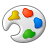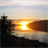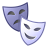# how do you calculate rotation angle for data driven pages

11648
12
10-04-2011 09:52 AMNew Contributor III
I am using data driven pages with a pre-defined strip map grid. I would like to add the required rotation angle to an attribute field in my index layer. I can't find a way to make this work. I've tried the Calculate Grid Convergence Angle tool but this gives values that don't make sense (all values appear to be between -2 and 2, when the real rotation is far greater than that in most cases). I can make this work if I use the program to create the strip map grid, but not if I use an existing grid layer.
Tags (2)
12 RepliesbyEsri Contributor
The Calculate Grid Convergence Angle tool calculates the angle for true north from the center of each polygon. If you use the Strip Map Index tool in the Data Driven Pages toolset to create your index features the angle will be populated automatically. If you already have an index feature layer you can use one of the COGO Report tools on the COGO toolbar to measure the angles. I believe the Angle Between Two Lines, or the Distance and Direction tool will give you the angle you need.Regular Contributor II
It's much harder than that! I frequently want to adjust stripmap angles after they have been created and it is very difficult to calculate the angle of a polygon. COGO tools are no use because they only handle lines. You would have to split the polygons back to lines and then you would still not have a useful angle.

I wrote my own Python script and used math.tan2(y/x) to get bearings, that then had to be normalised.

You could use the new tool in 10.x Data Management>Features>Maximum Bounding Geometry
and set the option "Add geometry" which includes a nice field MBG_orientation suitable to use, but of course it is now in a new featureclass with all your nice attributes trashed, so you would have to make sure you group by SMAP_NUM , join back and recalculate. Still a bit less work that my Python script. But here it is anyway in case it helps you.

`# UpdateAngle.py# assumes rectangular polygons# find bearing of longest side# might have trouble with up# 2 June 2011# Kim Ollivierimport arcgisscriptingfrom math import *import datetimegp = arcgisscripting.create(9.3)try:    layer = sys.argvexcept:    layer = "stripindex"    ws = 'e:/teararoa/nz/current.gdb'    gp.workspace = wsif not gp.Exists(ws):    print "ws",ws    raise ExceptionrotField = "smap_angle"if  not gp.ListFields(layer,rotField):    gp.AddField(layer,rotField,"FLOAT")def calcbearing(dPt):    '''    calculate bearing of longest side    '''    # points    pt1 = dPt    pt2 = dPt    pt3 = dPt    # lengths    l1 = hypot(pt2 - pt1,pt2 - pt1)    l2 = hypot(pt3 - pt2,pt3 - pt2)    # bearings    b1 = atan2(pt2 - pt1,pt2 - pt1)    b2 = atan2(pt3 - pt2,pt3 - pt2)    # print "test",round(l1),round(l2),degrees(b1),degrees(b2)    # print dPt    if l1 > l2 :        degree = degrees(b1)    else:        degree = degrees(b2)    bearing = 90.0 - degree    if  bearing > 90:        bearing = bearing - 180    if  bearing < -90:        bearing = bearing + 180    return bearingcur = gp.UpdateCursor(layer) ##,"ID = 1")row = cur.next()printwhile row:    feat = row.Shape    for partNum in range(feat.partCount) :        part = feat.getPart(partNum)        dPt = {}        n = 0        for pt in iter(lambda: part.next(),None): # stops at null pt            n+=1            dPt = (pt.X,pt.Y)    rotation = calcbearing(dPt)    row.setValue(rotField,rotation)    row.setValue("LastUpdate",datetime.datetime.now())    # print row.objectid,row.id,row.island,rotation #,dPt    cur.updateRow(row)    row = cur.next()del row,cur`

Next the challenge will be to write something that will rotate the feature from the attribute. Numpy will be useful here.New Contributor III

Has anyone found a Simple solution to this? I am experiencing the same problembyMVP Honored Contributor

I believe you will still have to rely on a custom python script, although using arcpy geometry objects will likely shorten Kim's script considerably. I'll try to follow up on this.byMVP Honored Contributor

Here is a 10.1 script you can use to calculate the data driven page rotation angle into a field called calcAngle, for an index feature class of rectangles.

`>>> import math... with arcpy.da.UpdateCursor("RECTANGLE_INDEX_FEATURE CLASS",["SHAPE@","calcAngle"]) as cursor:...    for row in cursor:...        points = []...        for part in row:...            for pnt in part:...                points.append(pnt)...        lines = []...        for i in range(1,3):...            lines.append(arcpy.Polyline(arcpy.Array([points,points[i-1]])))...        if lines.length > lines.length:...            firstShort = 0...        else:...            firstShort = 1...        dx = points[firstShort].X - points[firstShort+1].X...        dy = points[firstShort].Y - points[firstShort+1].Y...        ang = math.degrees(math.atan(dy/dx))...        row = 360-ang...        cursor.updateRow(row)`New Contributor II

Hi Darren,

This looks exactly like what I need however I cannot get the code to work for me. I am trying this in 10.3. I get the error message :Runtime error
Traceback (most recent call last):
File "<string>", line 6, in <module>
TypeError: 'float' object is not iterable

I changed the Feature class and field names to match my layer. ("Release_Grid",["Shape","calcAngle"]

Any suggestions?byMVP Honored Contributor

Make sure you use "SHAPE@", a special token for the actual geometry.New Contributor II

Got it! Thank you!!Occasional Contributor II

Thanks for Darren's script which works perfectly. However I found it requires the 4 points of the polygon be created in a certain order, which might be all right for index polygons created via DDP tool, but in our case we often got the index polygons from other source like CAD design where how it was created not always guaranteed. So I modified Darren's code a bit to allow the calculation work on polygons created in any direction. cheers,